Functions IF, AND, OR in Excel

In this example, I show you how to use IF, AND, OR functions together in the Excel worksheet. There is not only the one solution in logical tasks. You can always modify the formula in different ways.

Example

There are two exams in the table. Each student has to pass both of exams. Status is OK means that he passed. Status NOK means he did not pass.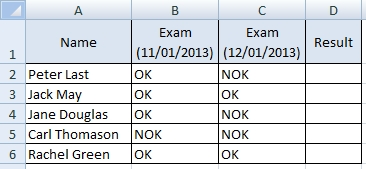Task: Create a function that evaluate if both exams are OK. If it’s true then Result is Y. If it’s not true then Result is N.

Solution by using functions IF, AND

We will focus on the first student and the result Y. When it occurs? The answer is: When the cells B2 and C2 are both set to OK. So, we create the function like this:

=IF(AND(B2=”OK”,C2=”OK”),”Y”,”N”)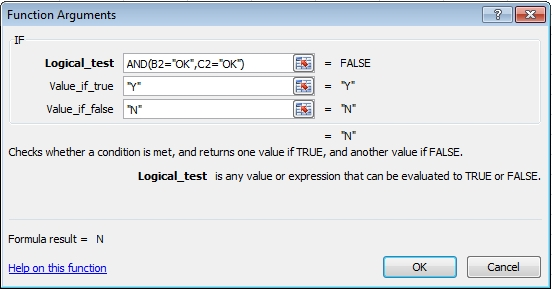The parameters OK, Y and N are text strings so they have to be in the quotation marks.

Function AND returns only TRUE/FALSE value.
- If all of the logical tests in the AND function are TRUE, the result is TRUE.
- If at least one of the logical tests is FALSE, the result is FALSE.

Solution by using functions IF, OR

Now, we examine the result N. It occurs when the cell B2 or C2 is set to NOK. The formula will be:

=IF(OR(B2=”NOK”,C2=”NOK”),”N”,”Y”)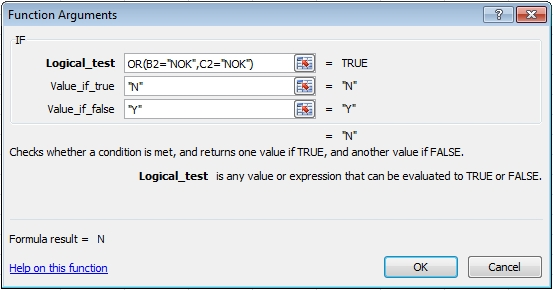Notice that the combination IF with AND is a logical opposite of the function IF with OR. Reverse is also true, OR is a logical opposite of AND.

Solution by using multiple IF

This is a little bit different way to solve the logical problem. We put one IF function into the other.

=IF(B2=”OK”,IF(C2=”OK”,”Y”,”N”),”N”)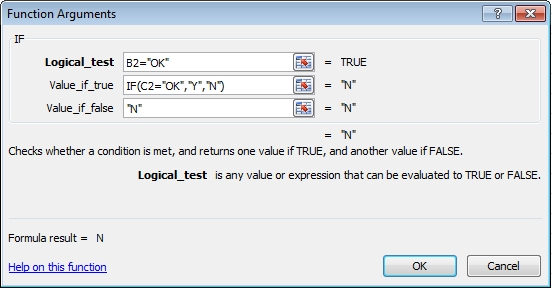The first IF function will evaluate B2=”OK”. If it’s FALSE then the result is N. If it’s TRUE we need the other IF function to evaluate C2=”OK”. If it’s TRUE the result is Y, if not then the result is N.

You can change this formula to check B2=”NOK”. The principle is the same but everything is inverted.

=IF(B2=”NOK”,”N”,IF(C2=”NOK”,”N”,”Y”))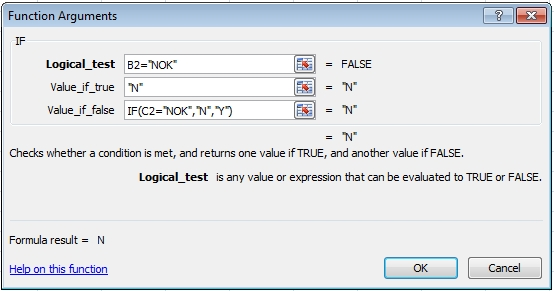Result

You can go by different ways but the result will be always the same and correct.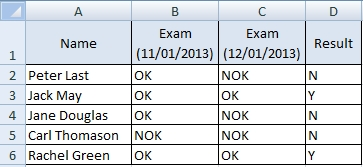- The formula is shorter (especially when you need to test more conditions).
- Sometimes, it is very hard to decide whether to use AND or OR.

- The formula is longer (especially when you need to test more conditions).
- In more complex task is easier to figure out the formula.

Useful tool – Evaluate Formula

This tool can help you to better understand the formula and find errors. Go to the menu Formulas >> Evaluate Formula.

Good luck and be patient. Logical formulas sometimes need more time to think through.

1.2.